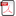### Derivation of Generalized Young’s Equation for Wetting of Cylindrical Droplets on Rough Solid Surface

•  Xiao-Song Wang

#### Abstract

The surface tension depends on the radius of curvature of the liquid-vapor interface. For nano-scale wetting phenomena of cylindrical droplets, we should consider the curvature effects of the surface tension and the line tension. However, previous works have not analyzed the influence of the curvature effects of the surface tension. In this paper, we discuss the influence of the curvature effects of the surface tension on the contact angles based the Kim-Lee-Han-Park equation. The hydrophilic wetting of cylindrical droplets on rough and chemically homogeneous non-deformable substrates were studied by methods of thermodynamics. A generalized Young’s equation for wetting of cylindrical droplets on chemically homogeneous and rough non-deformable substrates was derived based on the thermodynamic equilibrium conditions. This equation reduces to the Wenzel equation if we ignore the influence of line tension. For contact angles of cylindrical droplets with sufficiently large radii, a generalized Young’s equations were derived considering the curvature effects of the surface tension.

• Full Text:PDF
• DOI:10.5539/mer.v5n2p17This work is licensed under a Creative Commons Attribution 4.0 License.
• ISSN(Print): 1927-0607
• ISSN(Online): 1927-0615
• Started: 2011
• Frequency: semiannual